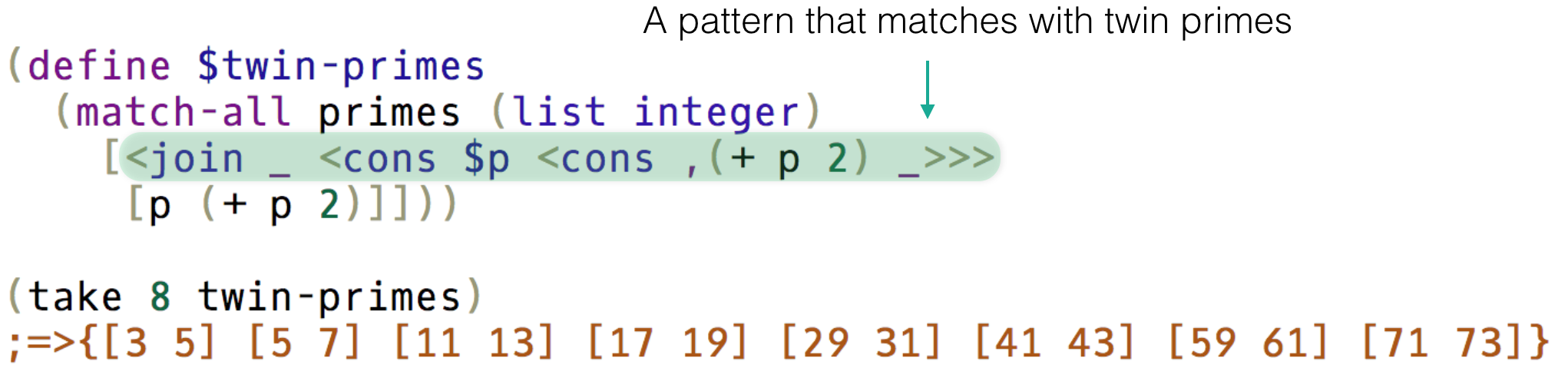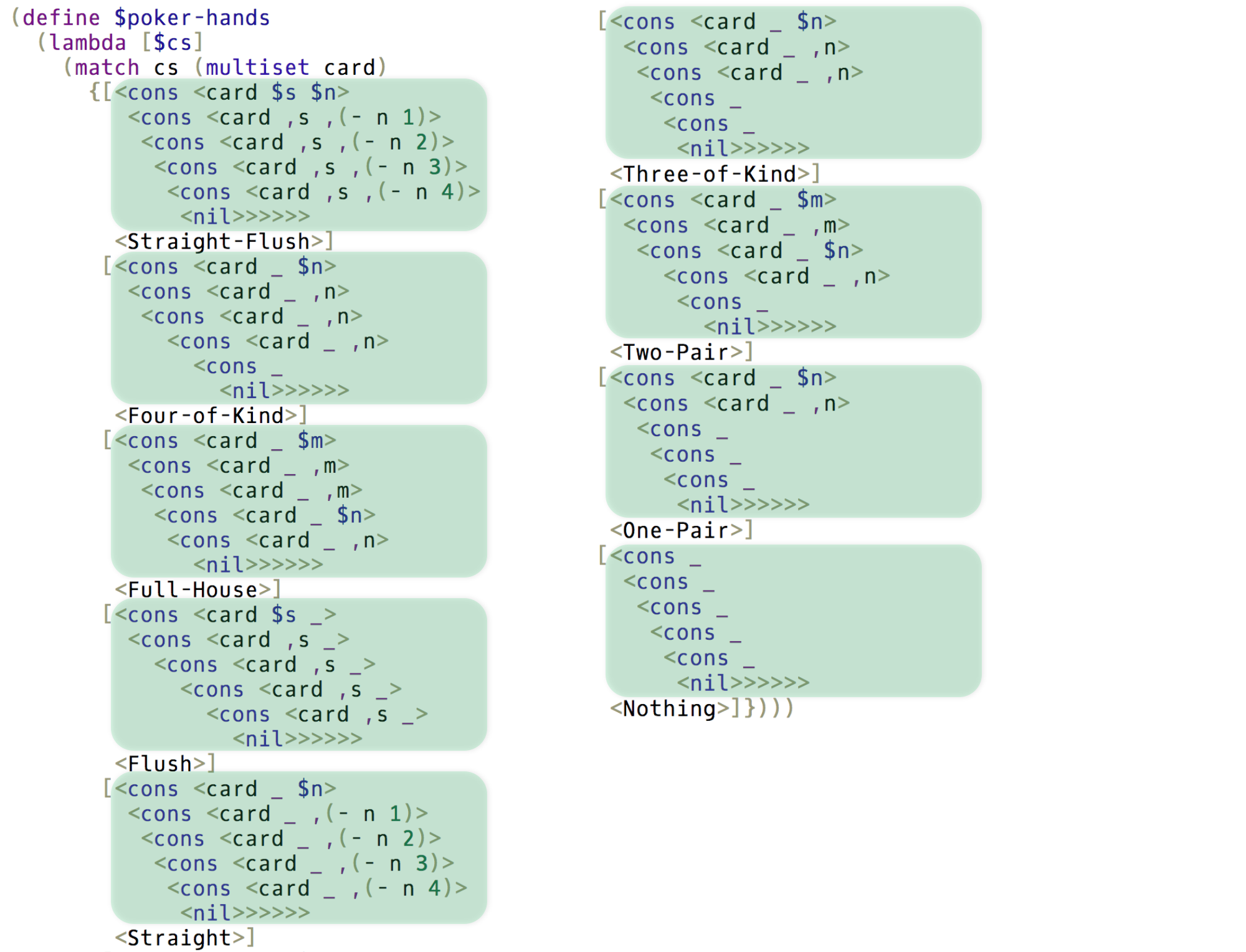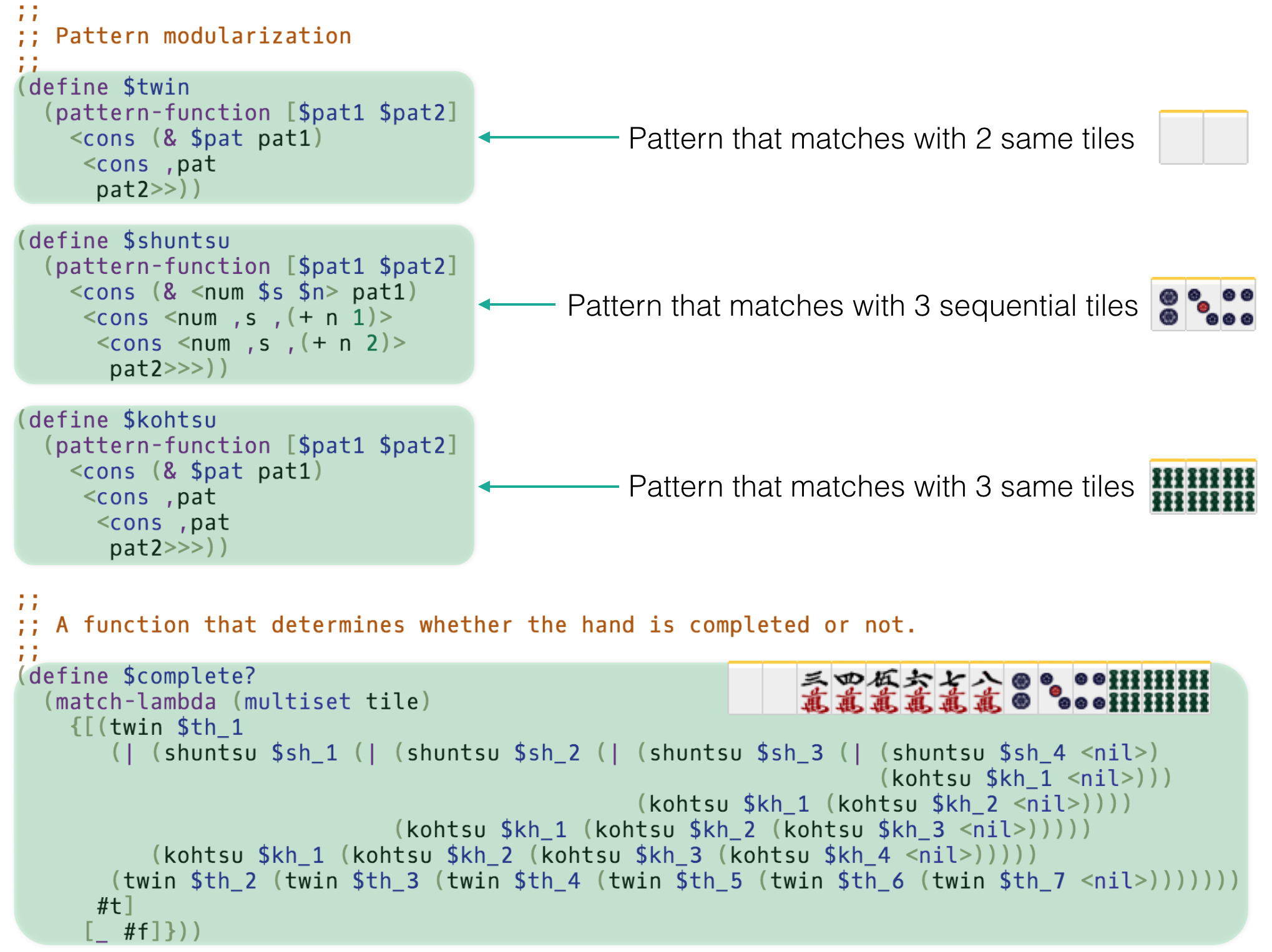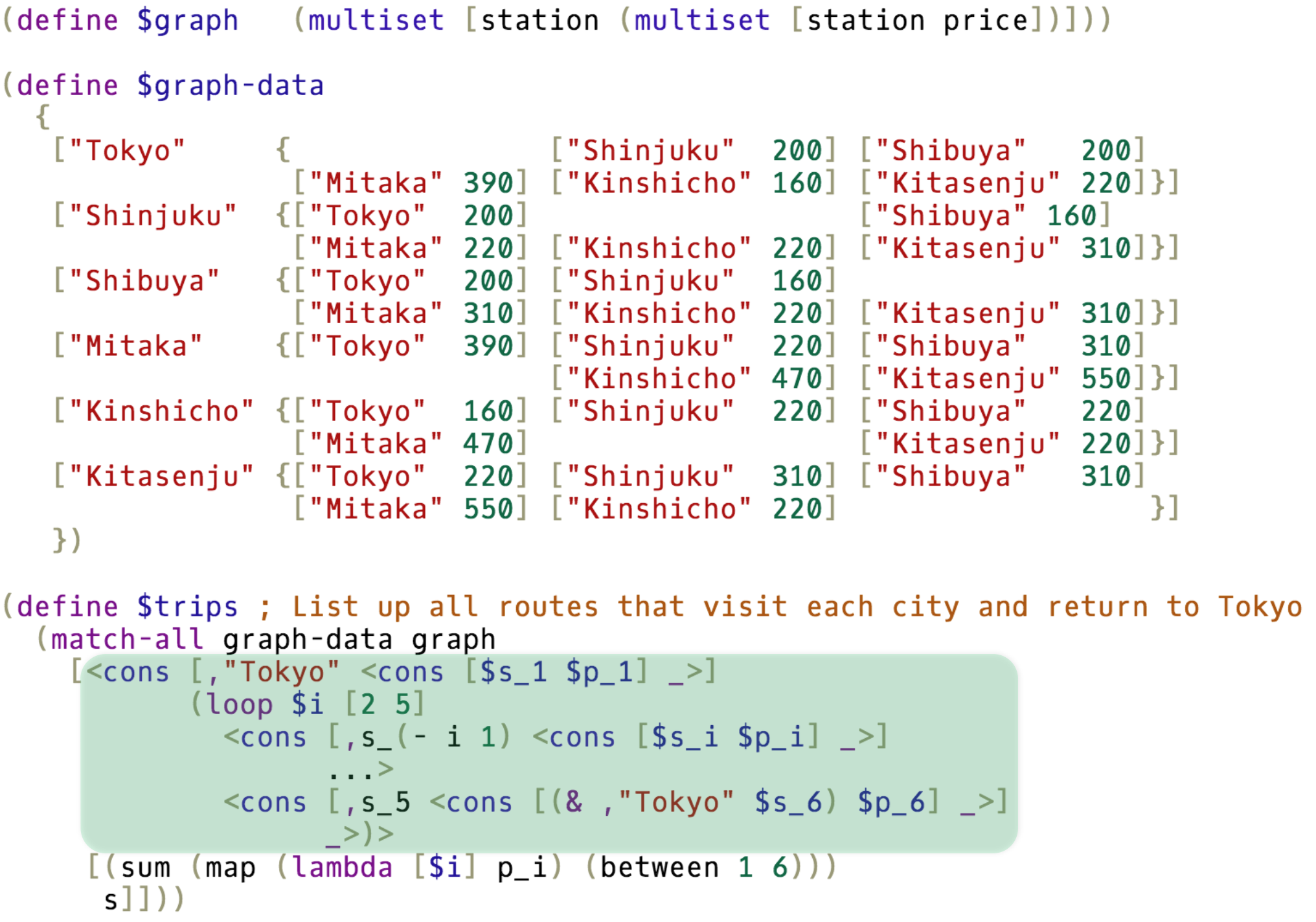# egison-mode Tag 3.9.2

Egison editing mode

Keywords
computer-algebra-system, differential-geometry, egison, functional-programming, pattern-matching, programming-language, tensor
MIT

# The Egison Programming Language

Egison is a functional programming language featuring its expressive pattern-matching facility. Egison allows users to define efficient and expressive pattern-matching methods for arbitrary user-defined data types including non-free data types such as lists, multisets, sets, trees, graphs, and mathematical expressions. This is the repository of the interpreter of Egison.

## Non-Linear Pattern Matching for Non-Free Data Types

We can describe non-linear pattern matching for non-free data types in Egison. A non-free data type is a data type whose data have no canonical form, a standard way to represent that object. For example, multisets are non-free data types because the multiset {a,b,b} has two other equivalent but literally different forms {b,a,b} and {b,b,a}. Expressive pattern matching for these data types enables us to write elegant programs.

### Twin Primes

We can use pattern matching for enumeration. The following code enumerates all twin primes from the infinite list of prime numbers with pattern matching!### Poker Hands

The following code is the program that determines poker-hands written in Egison. All hands are expressed in a single pattern.### Mahjong

We can write a pattern even against mahjong tiles. We modularize patterns to represent complex mahjong hands.### Graphs

We can pattern-match against graphs. We can write program to solve the travelling salesman problem in a single pattern-matching expression.Aren't these exciting? The pattern-matching facility of Egison is very powerful. We can use it for pattern matching also for graphs and tree-structures such as XML.

## Egison as a Computer Algebra System

As an application of Egison pattern matching, we have implemented a computer algebra system on Egison. The most part of this computer algebra system is written in Egison and extensible using Egison.

### Symbolic Algebra

Egison treats unbound variables as symbols.

``````> x
x
> (** (+ x y) 2)
(+ x^2 (* 2 x y) y^2)
> (** (+ x y) 10)
(+ x^10 (* 10 x^9 y) (* 45 x^8 y^2) (* 120 x^7 y^3) (* 210 x^6 y^4) (* 252 x^5 y^5) (* 210 x^4 y^6) (* 120 x^3 y^7) (* 45 x^2 y^8) (* 10 x y^9) y^10)
``````

We can handle algebraic numbers, too.

``````> (sqrt x)
(sqrt x)
> (sqrt 2)
(sqrt 2)
> (sqrt 4)
2
> (+ x (sqrt y))
(+ x (sqrt y))
``````

### Complex Numbers

The symbol `i` is defined to rewrite `i^2` to `-1` in Egison library.

``````> (* i i)
-1
> (* (+ 1 (* 1 i))  (+ 1 (* 1 i)))
(* 2 i)
> (** (+ 1 (* 1 i)) 10)
(* 32 i)
> (* (+ x (* y i))  (+ x (* y i)))
(+ x^2 (* 2 i x y) (* -1 y^2))
``````

### Square Root

The rewriting rule for `sqrt` is also defined in Egison library.

``````> (* (sqrt 2) (sqrt 2))
2
> (* (sqrt 6) (sqrt 10))
(* 2 (sqrt 15))
> (sqrt x)
(sqrt x)
> (* (sqrt (* x y)) (sqrt (* 2 x)))
(* x (sqrt 2) (sqrt y))
``````

### The 5th Roots of Unity

The following is a sample to calculate the 5th roots of unity.

``````> (q-f' 1 1 -1)
[(/ (+ -1 (sqrt 5)) 2) (/ (+ -1 (* -1 (sqrt 5))) 2)]
> (define \$t (fst (q-f' 1 1 -1)))
> (q-f' 1 (* -1 t) 1)
[(/ (+ -1 (sqrt 5) (sqrt (+ -10 (* -2 (sqrt 5))))) 4) (/ (+ -1 (sqrt 5) (* -1 (sqrt (+ -10 (* -2 (sqrt 5)))))) 4)]
> (define \$z (fst (q-f' 1 (* -1 t) 1)))
> z
(/ (+ -1 (sqrt 5) (sqrt (+ -10 (* -2 (sqrt 5))))) 4)
> (** z 5)
1
``````

### Differentiation

We can implement differentiation easily in Egison.

``````> (d/d (** x 3) x)
(* 3 x^2)
> (d/d (** e (* i x)) x)
(* i (** e (* i x)))
> (d/d (d/d (log x) x) x)
(/ -1 x^2)
> (d/d (* (cos x) (sin x)) x)
(+ (* -1 (sin x)^2) (cos x)^2)
``````

### Taylor Expansion

The following sample executes Taylor expansion on Egison. We verify Euler's formula in the following sample.

``````> (take 8 (taylor-expansion (** e (* i x)) x 0))
{1 (* i x) (/ (* -1 x^2) 2) (/ (* -1 i x^3) 6) (/ x^4 24) (/ (* i x^5) 120) (/ (* -1 x^6) 720) (/ (* -1 i x^7) 5040)}
> (take 8 (taylor-expansion (cos x) x 0))
{1 0 (/ (* -1 x^2) 2) 0 (/ x^4 24) 0 (/ (* -1 x^6) 720) 0}
> (take 8 (taylor-expansion (* i (sin x)) x 0))
{0 (* i x) 0 (/ (* -1 i x^3) 6) 0 (/ (* i x^5) 120) 0 (/ (* -1 i x^7) 5040)}
> (take 8 (map2 + (taylor-expansion (cos x) x 0) (taylor-expansion (* i (sin x)) x 0)))
{1 (* i x) (/ (* -1 x^2) 2) (/ (* -1 i x^3) 6) (/ x^4 24) (/ (* i x^5) 120) (/ (* -1 x^6) 720) (/ (* -1 i x^7) 5040)}
``````

### Tensor Index Notation

Egison supports tesnsor index notation. We can use Einstein notation to express arithmetic operations between tensors.

The method for importing tensor index notation into programming is discussed in Egison tensor paper.

The following sample is from Riemann Curvature Tensor of S2 - Egison Mathematics Notebook.

``````;; Parameters
(define \$x [|θ φ|])

(define \$X [|(* r (sin θ) (cos φ)) ; = x
(* r (sin θ) (sin φ)) ; = y
(* r (cos θ))         ; = z
|])

;; Local basis
(define \$e_i_j (∂/∂ X_j x~i))
e_i_j
;[|[|(* r (cos θ) (cos φ)) (* r (cos θ) (sin φ)) (* -1 r (sin θ)) |]
;  [|(* -1 r (sin θ) (sin φ)) (* r (sin θ) (cos φ)) 0 |]
;  |]_#_#

;; Metric tensor
(define \$g__ (generate-tensor 2#(V.* e_%1_# e_%2_#) {2 2}))
(define \$g~~ (M.inverse g_#_#))

g_#_#;[| [| r^2 0 |] [| 0 (* r^2 (sin θ)^2) |] |]_#_#
g~#~#;[| [| (/ 1 r^2) 0 |] [| 0 (/ 1 (* r^2 (sin θ)^2)) |] |]~#~#

;; Christoffel symbols
(define \$Γ_j_k_l
(* (/ 1 2)
(+ (∂/∂ g_j_l x~k)
(∂/∂ g_j_k x~l)
(* -1 (∂/∂ g_k_l x~j)))))

(define \$Γ~__ (with-symbols {i} (. g~#~i Γ_i_#_#)))

Γ~1_#_#;[| [| 0 0 |] [| 0 (* -1 (sin θ) (cos θ)) |] |]_#_#
Γ~2_#_#;[| [| 0 (/ (cos θ) (sin θ)) |] [| (/ (cos θ) (sin θ)) 0 |] |]_#_#

;; Riemann curvature tensor
(define \$R~i_j_k_l
(with-symbols {m}
(+ (- (∂/∂ Γ~i_j_l x~k) (∂/∂ Γ~i_j_k x~l))
(- (. Γ~m_j_l Γ~i_m_k) (. Γ~m_j_k Γ~i_m_l)))))

R~#_#_1_1;[| [| 0 0 |] [| 0 0 |] |]~#_#
R~#_#_1_2;[| [| 0 (sin θ)^2 |] [| -1 0 |] |]~#_#
R~#_#_2_1;[| [| 0 (* -1 (sin θ)^2) |] [| 1 0 |] |]~#_#
R~#_#_2_2;[| [| 0 0 |] [| 0 0 |] |]~#_#
``````

### Differential Forms

By designing the index completion rules for omitted indices, we can use the above notation to express a calculation handling the differential forms.

The following sample is from Curvature Form - Egison Mathematics Notebook.

``````;; Parameters and metric tensor
(define \$x [| θ φ |])

(define \$g__ [| [| r^2 0 |] [| 0 (* r^2 (sin θ)^2) |] |])
(define \$g~~ [| [| (/ 1 r^2) 0 |] [| 0 (/ 1 (* r^2 (sin θ)^2)) |] |])

;; Christoffel symbols
(define \$Γ_i_j_k
(* (/ 1 2)
(+ (∂/∂ g_i_k x~j)
(∂/∂ g_i_j x~k)
(* -1 (∂/∂ g_j_k x~i)))))

(define \$Γ~i_j_k (with-symbols {m} (. g~i~m Γ_m_j_k)))

;; Connection form
(define \$ω~i_j (with-symbols {k} Γ~i_j_k))

;; Curvature form
(define \$d
(lambda [%A]
!((flip ∂/∂) x A)))

(define \$wedge
(lambda [%X %Y]
!(. X Y)))

(define \$Ω~i_j (with-symbols {k}
(df-normalize (+ (d ω~i_j)
(wedge ω~i_k ω~k_j)))))
``````

### Egison Mathematics Notebook

Here are more samples.

## Comparison with Related Work

There are a lot of existing work for pattern matching.

The advantage of Egison is that it fulfills the following two requirements at the same time.

1. Efficient backtracking algorithm for non-linear pattern matching.
2. Extensibility of patterns.

Additionally, it fulfills the following requirements.

1. Polymorphism of patterns.
2. Pattern matching with infinitely many results.

## Installation

If you are using Linux, please install `libncurses-dev` at first.

``````% sudo apt-get install libncurses-dev # on Debian
``````

To compile Egison, you also need to install Haskell Platform.

After you installed Haskell Platform, run the following commands on the terminal.

``````% cabal update
% cabal install egison
``````

Now, you can try Egison.

``````% egison
Egison Version X.X.X(C) 2011-2014 Satoshi Egi
https://www.egison.org
Welcome to Egison Interpreter!
> ^D
Leaving Egison Interpreter.
``````

If you are a beginner of Egison, it would be better to install `egison-tutorial`.

``````% cabal update
% cabal install egison-tutorial
% egison-tutorial
Egison Tutorial Version 3.7.4 (C) 2013-2017 Satoshi Egi
Welcome to Egison Tutorial!
** Information **
We can use a 'Tab' key to complete keywords on the interpreter.
If we type a 'Tab' key after a closed parenthesis, the next closed parenthesis will be completed.
*****************
==============================
List of sections in the tutorial.
1: Calculate numbers
2: Basics of functional programming
3: Basics of pattern matching
4: Pattern matching against various data types
5: Symbolic computation
6: Differential geometry: tensor analysis
7: Differential geometry: differential forms
==============================
Choose a section to learn.
(1-7): 1
====================
We can do arithmetic operations with '+', '-', '*', '/', 'modulo' and 'power'.

Examples:
(+ 1 2)
(- 30 15)
(* 10 20)
(/ 20 5)
(modulo 17 4)
(power 2 10)
====================
>
``````

We can try it also online. Enjoy!

## Note for Developers

### How to Run Test

``````% cabal test
``````

### How to Profile the Interpreter

``````% sudo apt-get install haskell-platform-doc haskell-platform-prof
% cabal sandbox init
% cabal install --enable-profiling
% egison +RTS -p -RTS -l sample/sequence.egi
% cat egison.prof
``````

## Acknowledgement

I thank Ryo Tanaka, Takahisa Watanabe, Takuya Kuwahara, Kentaro Honda, and Mayuko Kori for their help to implement the interpreter.